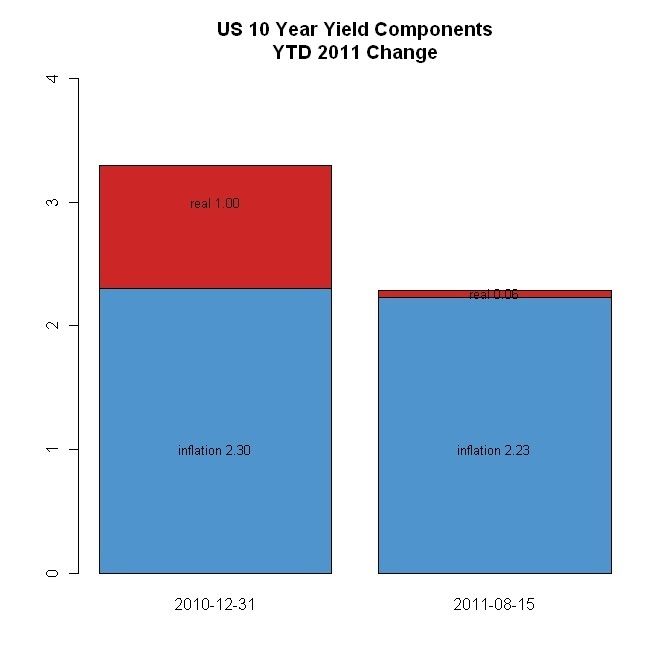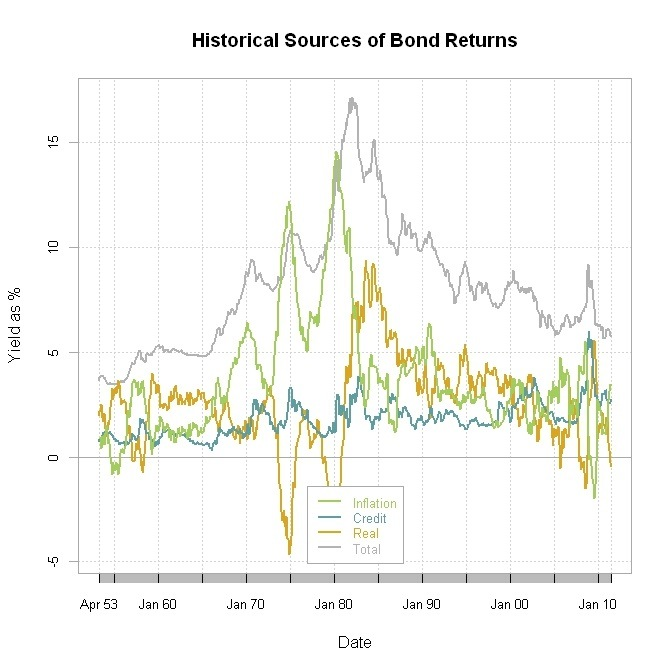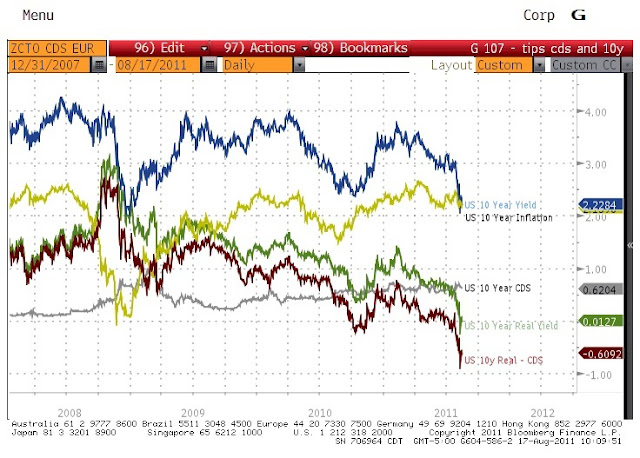## Thursday, August 25, 2011

### Mode vs Mean in Tactical Allocation

Let’s take Modest Modeest for Moving Average one step further and use it in a basic tactical allocation system using Vanguard funds.  THIS IS NOT INVESTMENT ADVICE AND VERY EASILY MIGHT CAUSE LARGE LOSSES.  VANGUARD FUNDS IMPOSE EARLY REDEMPTION FEES THAT RENDER THIS NOT FEASIBLE.

If we use US Equities (vfinx for S&P 500), International Developed (vgstx), and Emerging (veiex) to fill the 60% equities slot in a portfolio and then add 40% US Bonds (vbmfx), we can fairly closely resemble a typical balanced allocation.  Instead of buy and hold, let’s only buy and hold when each is above its respective 10 month mode, but sell whenever it falls below that mode.  The result is fairly attractive especially in terms of drawdown reduction.

Ultimately, to choose mode over mean, we should evaluate versus the Mebane Faber 10 month moving average system.  The results are far from conclusive, but both clearly beat the balanced benchmark in terms of drawdown.  Since I would think it is much easier to explain mean to clients, I would say that Mebane’s system wins in this very simple framework.  However, this is only start.  Please let me know any discoveries as you apply to a much more diverse set of allocation options.

`require(quantmod)require(modeest)require(PerformanceAnalytics)   start = "1990-12-31"end = Sys.Date()   #use Vanguard funds as proxy for asset classes#due to Vanguard fees for early redemption#these funds not appropriate for Tactical systemtckrs <- c("VFINX","VEIEX","VGSTX","VBMFX")getSymbols(tckrs, from=start, to=end, adjust=TRUE)for (i in 1:length(tckrs)) {	assign(tckrs[i],to.monthly(get(tckrs[i]))[,4])}   funds <- na.omit(merge(VFINX[,1],VEIEX[,1],VGSTX[,1],VBMFX[,1]))#get dates to include day so format is YYYY-MM-DDindex(funds) <- as.Date(index(funds))colnames(funds) <- tckrs   #get 1 month changefunds.roc <- ROC(funds,type="discrete",n=1)#add a balanced benchmark#20% of each equity fund for 60% total equities#40% of US bondsfunds.roc <- merge(funds.roc,	0.2*funds.roc[,1]+0.2*funds.roc[,2]+0.2*funds.roc[,3]+0.4*funds.roc[,4])funds.roc[1,5] <- 0colnames(funds.roc) <- c(colnames(funds),"Balanced")   #combine all the funds into one xtsfunds <- merge(funds,cumprod(1+funds.roc[,5]))#charts.PerformanceSummary(funds.roc,#	main="Vanguard Funds for US, Emerging, Intl Stocks and US Bonds#	with a 60/40 Balanced Allocation")   #set width to be 10 months similar to Mebane Faber 10 month moving averagewidth = 10#set up system.signal with same number of columns and rows as fundssystem.signal <- funds#loop through each column since I could not use apply with mode calculationfor (m in 1:NCOL(system.signal)) {	#another for loop to do the rolling mode since it also does not work with apply	for (n in width:NROW(system.signal[,m])) {		md <- mlv(funds[(n-width):n,m], method = "lientz", bw=0.4)		ifelse(n==width,			md.hist <- as.numeric(md),			md.hist <- rbind(md.hist,as.numeric(md)))	}	#make into nice xts	md.xts <- as.xts(as.data.frame(md.hist),		order.by=index(system.signal[(width):NROW(system.signal)]))	#put rolling modes into system.signal	system.signal[(width):NROW(system.signal),m] <- md.hist}   #jpeg(filename="mode chart.jpg",#	quality=100,width=6.25, height = 6.25,  units="in",res=96)plot.zoo(system.signal,main="Modes (lientz) of Funds",nc=1,	col=c("gray70","steelblue4","darkolivegreen4","goldenrod","purple"))#dev.off()   #difficult way to get 1 for in and 0 for out#but the ifelse gave me an index increasing order error#will use apply insteadsystem.signal <- funds > system.signalsystem.signal[,1:length(tckrs)] <- apply(system.signal[,1:length(tckrs)],MARGIN=2,FUN=as.numeric)ret.signal <- lag(system.signal,k=1) * funds.roc[,1:5]ret.compare <- merge(funds.roc[,4],	0.2*ret.signal[,1]+0.2*ret.signal[,2]+0.2*ret.signal[,3]+0.4*ret.signal[,4],	ret.signal[,5],	funds.roc[,5])colnames(ret.compare) <- c("VBMFX.Bonds","ModeSystem","ModeOnBalanced","Balanced")   #jpeg(filename="signal chart.jpg",#	quality=100,width=6.25, height = 6.25,  units="in",res=96)plot.zoo(system.signal,main="Signals (hsm) of Funds",nc=1,	col=c("gray70","steelblue4","darkolivegreen4","goldenrod","purple"))#dev.off()   #jpeg(filename="performance summary no average.jpg",#	quality=100,width=6.25, height = 6.25,  units="in",res=96)charts.PerformanceSummary(ret.compare,ylog=TRUE,	main="Performance of Tactical Approaches	with Balanced Benchmark",	colorset=c("gray70","steelblue4","darkolivegreen4","goldenrod","purple"),	cex.legend=1.2,lwd=2)#dev.off()     #now let's compare to Mebane Faber 10-month moving average strategy#will do in 1 line since there are multiple examples of how#to implement this in Rret.average <- lag(as.xts(apply(	funds > apply(funds[,1:5],MARGIN=2,FUN=runMean,10),MARGIN=2,as.numeric),order.by=index(funds)),k=1) * funds.rocret.compare <- merge(ret.compare,	0.2*ret.average[,1]+0.2*ret.average[,2]+0.2*ret.average[,3]+0.4*ret.average[,4])colnames(ret.compare) <- c(colnames(ret.compare)[1:(NCOL(ret.compare)-1)],"Faber10moAvg")   #jpeg(filename="performance summary with Faber average.jpg",#	quality=100,width=6.25, height = 6.25,  units="in",res=96)charts.PerformanceSummary(ret.compare,ylog=TRUE,	main="Performance of Tactical Approaches	with Balanced Benchmark and Mebane Faber 10mo Mov Avg",	colorset=c("gray70","steelblue4","darkolivegreen4","goldenrod","purple"),	cex.legend=1.2,lwd=2)#dev.off()`

Created by Pretty R at inside-R.org

## Wednesday, August 24, 2011

### Modest Modeest for Moving Average

I have no idea who originated the idea of using moving averages to determine entry and exit points in a trading system.  I do know that Mebane Faber (briefly discussed in Shorting Mebane Faber) has recently popularized the notion through his >75,000 download SSRN working paper

Faber, Mebane T., A Quantitative Approach to Tactical Asset Allocation (February 17, 2009). Journal of Wealth Management, Spring 2007. Available at SSRN: http://ssrn.com/abstract=962461

and his book

The general measures of central tendency are mean (average), median, and mode.  When I spotted the modeest package in my Planet R feed and then read When Are Averages Useless at ExploringDataBlog, I thought I should at least try all these mode options as a potential replacement to the Faber 10-month moving average.  I must confess that I do not understand all of these various mode options, but through the beauty of R I really don’t need to completely understand them to apply them.

AS ALWAYS THIS IS NOT INVESTMENT ADVICE.  THIS IS MERELY AN ILLUSTRATION AND MOST LIKELY WILL RESULT IN SIGNIFICANT LOSSES.

After some very basic tests, it appears hsm works most consistently best on equity indexes and even works on Crazy RUT.  These modes might offer an attractive alternative to the far more popular moving average.

`require(modeest)require(quantmod)require(PerformanceAnalytics)   getSymbols("^GSPC",from="1896-01-01",to=Sys.Date())GSPC.monthly <- to.monthly(GSPC)[,4]index(GSPC.monthly) <- as.Date(index(GSPC.monthly))   #width will represent number of months for modewidth = 10for (i in width:NROW(GSPC.monthly)) {	#not real proud of this code	#please feel free to comment with improvements	m.default <- mlv(GSPC.monthly[(i-width):i])	m.lientz <- mlv(GSPC.monthly[(i-width):i], method = "lientz", bw=0.01)	m.hrm <- mlv(GSPC.monthly[(i-width):i], method = "hrm", bw=0.01)	m.hsm <- mlv(GSPC.monthly[(i-width):i], method = "hsm",tie.action = "mean")	m.grenander <- mlv(GSPC.monthly[(i-width):i], method = "grenander", p = 0.5)	m.parzen <- mlv(GSPC.monthly[(i-width):i], method = "parzen", kernel = "gaussian")	m.tsybakov <- mlv(GSPC.monthly[(i-width):i], method = "tsybakov")	m.asselin <- mlv(GSPC.monthly[(i-width):i], method = "asselin")	m.vieu <- mlv(GSPC.monthly[(i-width):i], method = "vieu")   m.all <- c(as.numeric(m.default), as.numeric(m.lientz),		as.numeric(m.hrm), as.numeric(m.hsm), as.numeric(m.grenander),		as.numeric(m.parzen), as.numeric(m.tsybakov),		as.numeric(m.asselin), as.numeric(m.vieu))	ifelse(i==width, m.hist <- m.all, m.hist <- rbind(m.hist,m.all))}   m.xts <- as.xts(as.data.frame(m.hist),order.by=index(GSPC.monthly[(width):NROW(GSPC.monthly),]))m.xts <- merge(m.xts,runMean(GSPC.monthly,n=10),runMedian(GSPC.monthly,n=10))colnames(m.xts) <- c("default","lientz","hrm","hsm","grenander","parzen",	"tsybakov","asselin","vieu","mean","median")GSPC.mode <- merge(GSPC.monthly,m.xts)   #there has to be a much cleaner way to accomplish thissignal <- GSPC.mode[,2:NCOL(GSPC.mode)]ret <- GSPC.mode[,2:NCOL(GSPC.mode)]for (i in 1:NCOL(signal)) {	signal[,i] <- ifelse(GSPC.mode[,1] > GSPC.mode[,i+1],1,0)	ret[,i] <- lag(signal[,i],k=1) * ROC(GSPC.mode[,1],type="discrete",n=1)}ret <- merge(ret,	ROC(GSPC.mode[,1],type="discrete",n=1))   #jpeg(filename="modeest performance summary.jpg",#	quality=100,width=6.25, height = 6.25,  units="in",res=96)charts.PerformanceSummary(ret,ylog=TRUE,lwd=c(rep(2,9),rep(4,3)),	main="Modeest System Comparison with Mean and Median")#dev.off()   t(table.AnnualizedReturns(ret))   #jpeg(filename="modeest risk return.jpg",#	quality=100,width=6.25, height = 6.25,  units="in",res=96)chart.RiskReturnScatter(ret)#dev.off()`

Created by Pretty R at inside-R.org

## Tuesday, August 23, 2011

### Drawdown Visualization Supplement

As commented on Drawdown Visualization,

“Also, take a look at the example in ?chart.Event for another visualization of drawdowns, in this case stacking them for comparison.”

the PerformanceAnalytics package offers a very helpful look at the path of drawdowns that we can apply to financial and economic time series.  Here is the result applied to the Dow Jones Industrial Average since 1896.

1929-1954 really messes this chart up, so let's limit the recovery to 750 days to get a better visualization.

I thought it would be even more fun to apply this example to the GDP series.  This could be extended to all sorts of interesting economic data—first in my mind are industrial production and consumer spending.

`require(quantmod)require(PerformanceAnalytics)   getSymbols("DJIA",src="FRED")getSymbols("DJTA",src="FRED")getSymbols("DJUA",src="FRED")   DJ <- merge(DJIA,DJTA,DJUA)   #DJ.yearly <- DJ[endpoints(DJ, on="years", k=1),]DJ.roc <- ROC(DJ,n=1,type="discrete")     #another method of viewing drawdowns#this is really easy with PerformanceAnalytics#here is how to apply the very nice example#provided in the documentation   DJ.draw = Drawdowns(DJ.roc[,1])drawdowns = table.Drawdowns(DJ.roc[,1])                          #jpeg(filename="DJIA worst drawdown event chart.jpg",# quality=100,width=6.25, height = 6.25,  units="in",res=96)chart.Events(DJ.draw, dates = drawdowns\$Trough, prior=max(na.omit(drawdowns\$"To Trough")), post=max(na.omit(drawdowns\$Recovery)), lwd=2, colorset=redfocus, legend.loc=NULL, main = "DJIA Worst Drawdowns")#dev.off()#drawdown is so long in 1929 that it messes up the chart#let's limit this to two years or 750 trading days#jpeg(filename="DJIA worst drawdown event chart with limit.jpg",# quality=100,width=6.25, height = 6.25,  units="in",res=96)chart.Events(DJ.draw, dates = drawdowns\$Trough, prior=max(na.omit(drawdowns\$"To Trough")), post=min(750,max(na.omit(drawdowns\$Recovery))), lwd=2, colorset=redfocus, legend.loc=NULL, main = "DJIA Worst Drawdowns with Recovery Limit at 750 Days")#dev.off()   #now let's do it for GDPgetSymbols("GDP",src="FRED")GDP.roc <- ROC(GDP,type="discrete",n=1)GDP.draw = Drawdowns(GDP.roc[,1])drawdownsGDP = table.Drawdowns(GDP.roc[,1])                          #jpeg(filename="GDP worst drawdown event chart.jpg",# quality=100,width=6.25, height = 6.25,  units="in",res=96)chart.Events(GDP.draw, dates = drawdownsGDP\$Trough, prior=max(na.omit(drawdownsGDP\$"To Trough")), post=max(na.omit(drawdownsGDP\$Recovery)), lwd=2, colorset=redfocus, legend.loc="bottomright",xlab="Quarters +/- Drawdown", main = "US GDP Worst Drawdowns")#dev.off()`

Created by Pretty R at inside-R.org

## Monday, August 22, 2011

### Drawdown Visualization

Drawdown is my favorite measure of risk.  It picks up extended autocorrelated pain often not seen in risk measures, and best illustrates frustration, panic, and loss of confidence (Drawdown Control Can Also Determine Ending Wealth).  I thought I should try some new ways to see it in R.  This first graph uses the zoo package to show 20% drawdowns in light pink and 40% drawdowns in darker pink.

PerformanceAnalytics makes drawdown graphs and overlays very easy, but for some reason I got an error in findDrawdowns, so I instead used the table.Drawdowns function to see the 5 worst drawdowns.

`require(quantmod)require(PerformanceAnalytics)   getSymbols("DJIA",src="FRED")getSymbols("DJTA",src="FRED")getSymbols("DJUA",src="FRED")   DJ <- merge(DJIA,DJTA,DJUA)   #DJ.yearly <- DJ[endpoints(DJ, on="years", k=1),]DJ.roc <- ROC(DJ,n=1,type="discrete")DJ.draw = Drawdowns(DJ.roc)   #jpeg(filename="DJ plot with Drawdowns zoo.jpg",# quality=100,width=6.25, height = 6.25,  units="in",res=96)plot.zoo(log(DJ),plot.type="single", col=c(2,3,4),ylab="log Price",xlab=NA,main="Dow Jones Indexes")rgb <- hcl(c(0, 0, 260), c = c(100, 0, 100), l = c(50, 90, 50), alpha = 0.3)xblocks(index(DJ),as.vector(DJ.draw[,1] < -0.20),col = rgb)xblocks(index(DJ),as.vector(DJ.draw[,1] < -0.40),col = rgb)legend("topleft",inset=0.05,colnames(DJ),fill=c(2,3,4),bg="white")#dev.off()   #if we want to add linear models#abline(lm(log(coredata(DJ[,1]))~as.Date(index(DJ))),col=2)#abline(lm(log(coredata(DJ[,2]))~as.Date(index(DJ))),col=3)#abline(lm(log(coredata(DJ[,3]))~as.Date(index(DJ))),col=4)     #another method of viewing drawdowns#this is really easy with PerformanceAnalytics#but for some reason I am getting an error#with findDrawdowns#will just shade worst drawdowns until I figure it outdrawdowns <- table.Drawdowns(DJ.roc[,1])drawdowns.dates <- cbind(format(drawdowns\$From),format(drawdowns\$To))drawdowns.dates[is.na(drawdowns.dates)] <- format(index(DJ.roc)[NROW(DJ.roc)])# to get in proper list format# thanks http://stackoverflow.com/questions/6819804/how-to-convert-a-matrix-to-a-list-in-rdrawdowns.dates <- lapply(seq_len(nrow(drawdowns.dates)), function(i) drawdowns.dates[i,])   #jpeg(filename="DJ plot with Drawdowns chart TimeSeries.jpg",# quality=100,width=6.25, height = 6.25,  units="in",res=96)chart.TimeSeries(DJ,ylog=TRUE, period.areas = drawdowns.dates,period.color = rgb, colorset=c(2,3,4), legend.loc="topleft", main = "Dow Jones Indexes" )#dev.off()#or even fancier#jpeg(filename="DJ plot with Drawdowns chart PerformanceSummary.jpg",# quality=100,width=6.25, height = 8.25,  units="in",res=96)charts.PerformanceSummary(DJ.roc,ylog=TRUE, period.areas = drawdowns.dates,period.color = rgb, colorset=c(2,3,4), legend.loc="topleft", main = "Dow Jones Indexes" )#dev.off()`

Created by Pretty R at inside-R.org

## Wednesday, August 17, 2011

### Real Squeeze

Real yields even out to 10 years have now been competely squeezed. Either bond investors need to accept even worse negative real yields or deflation needs to get ugly for additional price returns from here. If deflation is the outcome, then shorts in stocks and commodities will be much more rewarding than long bonds.From TimelyPortfolio

R code:

require(quantmod)

getSymbols("DGS10",src="FRED")
getSymbols("DFII10",src="FRED")

barplot(rbind(merge(DGS10["2010-12-31",]-DFII10["2010-12-31",],DFII10["2010-12-31",]),
merge(DGS10[NROW(DGS10),]-DFII10[NROW(DFII10),],DFII10[NROW(DFII10),])),
col=c("steelblue3","firebrick3"),
main="US 10 Year Yield Components
YTD 2011 Change",
ylim=c(0,4))
text(y=c(coredata(DGS10["2010-12-31",])-.3,coredata(DFII10["2010-12-31",]),
coredata(DGS10[NROW(DGS10),])-.02,coredata(DFII10["2010-12-31",])),
x=c(0.7,0.7,1.9,1.9),
c(paste("real",sprintf("%.2f",coredata(DFII10["2010-12-31",])),sep=" "),
paste("inflation",sprintf("%.2f",coredata(DGS10["2010-12-31",]-DFII10["2010-12-31",])),sep=" "),
paste("real",sprintf("%.2f",coredata(DFII10[NROW(DFII10),])),sep=" "),
paste("inflation",sprintf("%.2f",coredata(DGS10[NROW(DGS10),]-DFII10[NROW(DFII10),])),sep=" ")),
cex=0.8)

### -1% Guaranteed Real Real Return! Yummy??

If we’re cooking up a bond return, we have access to 3 ingredients: inflation, credit, and real. Historically, the recipe looks like this (as described in Historical Sources of Bond Returns).

0-5 parts inflation
+ 1-2 parts credit
+ 1-3 parts real

and the chart looks like this.From TimelyPortfolio

In the good old days credit did not apply to U S Treasuries, but let’s just assume that given the current situation that there is a 1.4% chance of a loss of 50% due to credit (equivalent to the current CDS price on 10y US Treasuries of 0.70%). The inflation component should not include this credit risk compensation, so for US Treasuries with no credit component, this number must be included in the real component. When US 10y TIPS yield 0% but even worse -0.25% last week, that means investors find -1% real real returns a yummy recipe.

I think I need to look elsewhere for opportunity.From TimelyPortfolio

Access to the US 10y CDS for everybody is available at Bloomberg.

R code:

```require(quantmod)
require(PerformanceAnalytics)   getSymbols("GS10",src="FRED") #load 10yTreasury
getSymbols("BAA",src="FRED") #load Corporate for credit
getSymbols("CPIAUCSL",src="FRED") #load CPI for inflation    bondReturnSources<-na.omit(merge(ROC(CPIAUCSL,12,type="discrete")*100,
BAA-GS10,GS10-ROC(CPIAUCSL,12,type="discrete")*100))
bondReturnSources<-merge(bondReturnSources,
bondReturnSources[,1]+bondReturnSources[,2]+bondReturnSources[,3]) #add for total
colnames(bondReturnSources)<-c("Inflation","Credit","Real","Total")   chart.TimeSeries(bondReturnSources,legend.loc="bottom",
main="Historical Sources of Bond Returns",
ylab="Yield as %",

Created by Pretty

## Tuesday, August 16, 2011

### ttrTests Experimentation

I was intrigued by the CRAN update on a package ttrTests, especially since quantstrat is not built for backtesting system parameters (not true since quantstrat offers parameter testing and I will demonstrate in later post) and analyzing system performance as I mentioned in A Quantstrat to Build On Part 6.  ttrTests offers a nice start to my ideal setup for system development, testing, and reporting.  In upcoming posts, I hope to build some functionality on top of ttrTests to accomplish my objectives.

I proposed a basic counting system in A Quantstrat to Build On Part 6, but randomly assigned a 50 week (250 days or 1 trading year) parameter to the count.  With the help of ttrTests and a couple of index series from Yahoo!Finance, let’s see what parameters work best.  Then we can aggregate and graph to visualize the results.  As always, THIS IS NOT INVESTMENT ADVICE, and I welcome comments and suggestions.

`#let's define our silly countupdown function#as a sample of a custom ttr ruleCUD <- function(x,params=50,...) {	#CUD takes the n-period sum of 1 (up days) and -1 (down days)	temp <- ifelse(runSum(ifelse(ROC(x,1,type="discrete") > 0,1,-1),params)>=0,1,0)	#replace NA with 0 at beginning of period	temp[is.na(temp)] <- 0	temp}   require(ttrTests)require(quantmod)require(lattice)require(reshape2)require(PerformanceAnalytics)   #defaults functions is overridden by ggplot2 and plyr if loaded#and will cause problems if you want to use ttrTests concurrently   tckrs <- c("GSPC","RUT","N225","GDAXI","DJUBS")   for (i in 1:length(tckrs)) {	getSymbols(paste("^",tckrs[i],sep=""),from="1896-01-01",to=Sys.Date())	test_price <- as.vector(get(tckrs[i])[,4])	#do parameter tests but plot=FALSE	#we will plot later	#if you want plot=TRUE make sure you add dev.new() here	param_results <- paramStats(x=test_price, ttr = CUD, start = 20, nSteps = 30, stepSize = 10,		restrict = FALSE, burn = 0, short = FALSE, condition = NULL,		silent = TRUE, TC = 0.001, loud = TRUE, plot = FALSE, alpha = 0.025,		begin = 1, percent = 1, file = "", benchmark = "hold")	#get excess returns and add to matrix	ifelse(i==1,param_all <- param_results[],		param_all <- cbind(param_all,param_results[]))	#get best parameter and add to matrix	ifelse(i==1,param_best <- param_results[],		param_best <- rbind(param_best,param_results[]))}rownames(param_best) <- tckrsprint(param_best)   param_all <- cbind(param_results[],param_all)#fix rownames and colnames for param_allcolnames(param_all) <- c("parameters",tckrs)   df <- as.data.frame(param_all)df.melt <- melt(df,id.vars=1)colnames(df.melt) <- c("parameters","index","excessreturn")param_plot <- xyplot(excessreturn~parameters,group=index,data=df.melt,	auto.key=TRUE,type="l",main="Excess Returns by Parameter")#jpeg(filename="excess return by parameter.jpg",	quality=100,width=6.25, height = 6.25,  units="in",res=96)print(param_plot)#want to add points for max but unsure how currently#df.melt[which(df.melt\$parameters==param_best[1,] & df.melt\$index==rownames(param_best) ),3]dev.off()   #get performance summary for the best parametersfor (i in 1:length(tckrs)) {	dev.new()	#jpeg(filename=paste(tckrs[i],"performance summary.jpg",sep=""),	#	quality=100,width=6.25, height = 6.25,  units="in",res=96)	ret <- merge(lag(CUD(get(tckrs[i])[,4],		coredata(param_best),k=1))*ROC(get(tckrs[i])[,4],type="discrete", n=1),		ROC(get(tckrs[i])[,4],type="discrete", n=1))	colnames(ret)<-c(paste(tckrs[i]," CUD System",sep=""),tckrs[i])	charts.PerformanceSummary(ret,ylog=TRUE)	#dev.off()	ret[1,]<-0	price_system <- merge(get(tckrs[i])[,4],		lag(CUD(get(tckrs[i])[,4],		coredata(param_best),k=1))*get(tckrs[i])[,4],		cumprod(1+ret[,1])*coredata(get(tckrs[i]))[coredata(param_best),4])	price_system[which(price_system[,2]==0),2] <- NA	colnames(price_system) <- c("Out","In","System")   dev.new()	#jpeg(filename=paste(tckrs[i],"entry analysis.jpg",sep=""),	#	quality=100,width=6.25, height = 6.25,  units="in",res=96)	chartSeries(price_system\$System,theme="white",log=TRUE,up.col="black",		yrange=c(min(price_system[,c(1,3)]),max(price_system[,c(1,3)])),		TA="addTA(price_system\$Out,on=1,col=2);		addTA(price_system\$In,on=1,col=3)",		name=paste(tckrs[i]," Linear Model System",sep=""))	#dev.off()}`